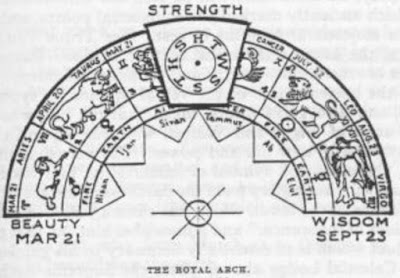## Cipher## Apr 28, 2017

### Masonic Arch + 9/11

Everyone that is even remotely familiar with Freemasonic symbolism will immediately recognize the Masonic Arch but there's a very special number showing up when we measure half of the zodiac wheel like thisFrom Spring Equinox (3/21) to Autumnal Equinox (9/23) is 187 days, this is one of the extremely significant numbers that seems to appear everywhere (also it is a very special number due to it's properties, factorization - 11x17 = 187 (187th prime number is 1117)

Bible begins with 5 books of Torah (the "law" as it is often translated), also Torah is 187 chapters long
Five books of Torah = 6+9+22+5 + 2+15+15+11+19 + 15+6 + 20+15+18+1+8 = 187 (Ordinal)
Aslo Aleister Crowley made his own ALW (LAW) gematria cipher and if you think about his teachings it's all based around Law.
The order & value of the letters in the ALW Cipher are derived from the grid superimposed on one of the pages of manuscript of Liber AL on which this verse appears (sheet 16 of Chapter III).
English Alphabet = 25+14+11+2+23+5+4 + 1+2+26+4+1+20+25+24 = 187 (ALW)

418 is a number connecting to entity Crowley encountered that is called "Aiwass", on the cover of one of his books you can see XCIII = 418 (93 = 418)
There's a lot more to be said about 187 but I don't wanna get sidetracked too much

So when I started thinking about importance of astrology to these people the first thing I measured from was 9/11 to Spring Equinox and Winter Solstice in 20019/11 attack lasted for 102 minutes, there was a 3/11/2004 Madrid Bombing 911 days after 9/11/2001 and we have 14 weeks and 4 days (144 is a very special number related to Time)
Madrid Train bombings = 4+1+4+9+9+4 + 2+9+1+9+5 + 2+6+4+2+9+5+7+10 = 102 (Single Reduction)One of the "airplanes" that hit the buildings was Flight 175 which was the most mysterious number to me and measuring from Spring Equinox I think we found the answer